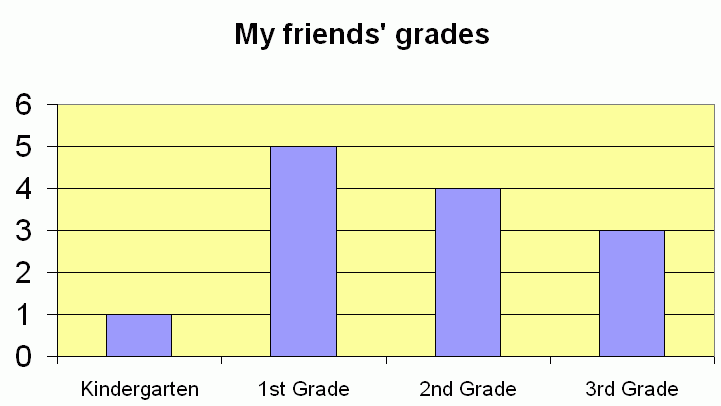SEARCH HOMEMath Central Quandaries & QueriesQuestion from Anthony's mom: we have a graph: Kindergarten has 1 1st has 5 2nd has 4 3rd has 3 is the mode "1st grade" because there are more children in that grade? 39 yr old mom isn't much help with 2nd grade math???Hi there.

I think perhaps your graph looks something like this:We have an online mathematics glossary that you can use to look up words like mode. It says:

 M mean in statistics, the measure of central tendency calculated by adding all the values and dividing the sum by the number of values. (Often referred to as the average.) median statistical the measure of central tendency that is in the middle when the values are arranged in order of size. If there is an even number of data items, the median is the mean of the middle two. of a triangle a line segment from any vertex of a triangle to the midpoint of the opposite side. mode the value that appears most frequently in a set of data.

So you are right. The most frequent grade is 1st Grade, so that is the mode.

The Median would be the middle value in the list when each person is listed in order of grade: